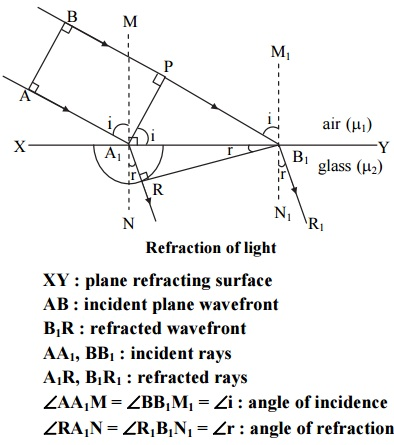# Explain refraction of light on the basis of wave theory. Hence prove laws of refraction .

##### Laws of refraction

Ratio of velocity of light in rarer medium to velocity of light in denser medium is a constant called refractive index of denser medium w.r.t. rarer medium. The incident rays, refracted rays and normal lie in the same plane. Incident ray and refracted ray lie on opposite sides of normal.

##### Explanation:

Phenomenon of refraction can be explained on the basis of wave theory of light.1. Let XY be the plane refracting surface separating two media air and glass of respectively. indices μ1 and μrefractive.
2. A plane wave front AB is advancing obliquely towards XY from air. It is bounded by rays AA1 and BB1 which are incident rays.
3. When ‘A’ reaches ‘A1’, then ‘B’ will be at ‘P’. It still has to cover distance PB1 to reach XY.
4. According to Huygens’ principle, secondary wavelets will originate from A1 and will spread over a hemisphere in glass.
5. All the rays between AA1 and BB1 will reach XY and spread over the hemispheres of increasing radii in glass. The surface of tangency of all such hemispheres is RB1. This gives rise to refracted wave front B1R in glass.
6. A1R and B1R1 are refracted rays.
7. Let c1 and c2 be the velocities of light in air and glass respectively.
8. . At any instant of time ‘t’, distance covered by incident wavefront from P to B1 = PB1 = c1t Distance covered by secondary wave from A1 to R = A1R = c2t.

What do you mean by polarisation ?